Electric Field & Potential

# Electric Field & Potential - Notes | Study Electromagnetic Fields Theory (EMFT) - Electrical Engineering (EE)

 1 Crore+ students have signed up on EduRev. Have you?

In this part of  Electrostatics, we will deal with properties of charges that are not in motion. In this lecture we will introduce you to the concept of an electric field and the potential associated with such a field.

The basic principle of electrostatics is based on the fact the electric charges attract or repel other charges depending on their relative signs and the law of force is given by Coulomb’s law.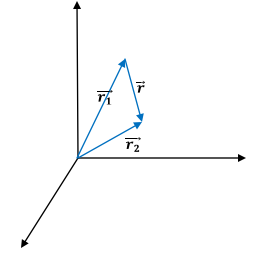The form of the law does not depend on the choice of origin. In the above figure, the force on the charge q2 located at the position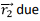to a charge  q1 located at the position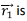is proportional to the product of the charges q1 and q2 and is inversely proportional to the square of the distance r between the charges. In vector form, the form of Newton’s law is written as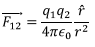Where we have assumed that the charges are interacting in vacuum with no medium between them. How things get modified if there is material medium will be discussed later in this course. The constant of proportionality in this case is written as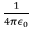in SI units. “permittivity of free space”. It has value close to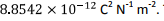The combination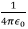in which it  appears in electromagnetic theory has a value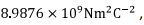, but is frequently approximated as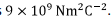We may note a few things about Coulomb’s law. If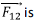the force on charge q2 due to the charge q1, by Newton’s third law, the force on the charge q1 due to q2 is equal and opposite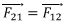The force is a “long range force” i.e. a force which does not become zero excepting at infinite distances. The nature of the force is “inverse square” in common with gravitational force between two masses. The force is called a “central force” whose magnitude depends on the distance between the objects and is directed along the line joining them.

Four Fundamental Forces in Nature

Before we begin, we would like to briefly talk about forces that exist in nature. There are basically four distinct types of forces, which go by the name “fundamental forces”. The gravitational force, which is the weakest of them all and is responsible for keeping the solar system with all its planets together is, like the electromagnetic force, is a central force and follows inverse square law. It is also a long range force. Its relative strength compared to the strong nuclear force is 10-39 . Stronger than the gravitational force but is third in the hierarchy of weakness is the “weak nuclear force’ which is responsible for processes such as beta decay of nuclei. It has a an extremely short range 10-18m, shorter than the dimension of a nucleon and a relative strength of 10-6.

The electrostatic force with which we are concerned with in this course is characterized by a relative strength given by a fundamental constant, known as the fine structure constant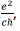is about 1/137 i.e. less than 1% of the strong nuclear force. As has been mentioned earler, the force is long ranged. In the above expression for the fine structure constant, e is the electronic charge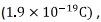c the speed of light in vacuum, which is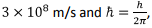is defined in terms of the Planck’s constant h.

The strongest of the forces is simply known as the “strong force” . It is the force which keeps the protons and neutrons bound to one another inside the nucleus. It is charge independent (i.e. the force is the same between a pair of protons or a pair of neutrons or that between a proton and neutron) and has a range which is of the order of nuclear dimension, viz 10-15m, which is also known as a “Fermi”.

What is the mechanism of interaction between two objects which are not in physical contact with each other? If we go from classical physics to quantum field theory (a subject which we do not need to go into), it transpires that two objects interact by continuously exchanging particles known as “bosons”. Incidentally, the name “boson” is after the celebrated Indian scientist Satyendra Nath Bose. It also turns out that if the force between two objects has finite range then the exchanged bosons have mass. On the other hand in case of long range forces the bosons are massless. In case of electromagnetic force, the exchanged particles are quanta of light known as “photons”, usually represented by the Greek letter γ.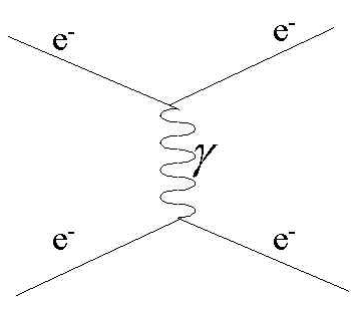Even with this, things are fine as long as the charges are static. Suppose two static charges are interacting via inverse square law. If now one of the charges moves. How does the force “instantly” change to correspond to the square of the new distance between the charges? We know from the special theory of relativity that no information can be transmitted with a speed greater than the speed of light in vacuum. Thus the principle of instantaneous change in the force law to reflect movement of one of the charges, i.e. the principle of “action at a distance”, is not consistent with the special theory of relativity. This is where concept of a field comes to our rescue. To get a very crude (and incorrect) picture, suppose we imagine that each charged particle is associated with a medium (not a material medium) which is tightly bound to it. When we talk about interaction of one charge with the other, it is the interaction between the second charge with the medium associated with the first. When the first charge moves, the medium gets deformed and enables the change to take place.
Taking a cue from this crude picture, we say that with every object (in our case a charged particle), a “field” is associated. When the object moves, the field changes and this information propagates with the speed of light, consistent with the special theory of relativity. This field in our case is a “vector field” known as the “electric field”. How does one define an electric field due to a charge q? Consider a “test charge” qt on which this charge q exerts a force. It is necessary that qt is taken infinitesimally small. This is because, qt itself being an electric charge will have its own electric field which will alter the field due to the charge q. So we require that the charge qt be so small it it should not significantly alter the field due to the charge q. We define the electric field due to the charge q,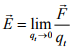Since we define it in the limit of vanishing test charge, the definition does not depend on qt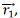In the above definition, the source of the electric field is arbitrary. If the field is due to a point charge q1 located at the position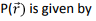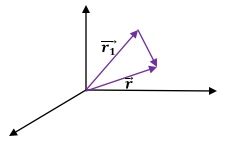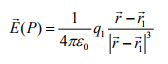Superposition Principle :

If the source of the electric field is due to multiple charges, the field is simply the vector sum of the fields due to each field. This is known as the superposition principle.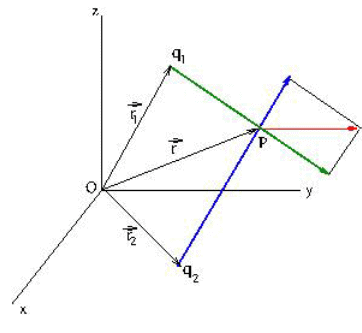This is illustrated in the figure above where the field at P located at position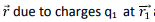and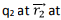P is shown as the vector sum of the fields due to each of the charges. This can be generalized to the case of multiple charges,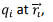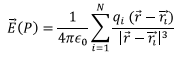We can extend this to the case of a continuous charge distribution,

Line, Surface and Volume Charges :

Let us consider charges distributed over an arbitrary curve. If we take a length element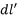along the curve, the amount of charge on this element is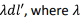is the linear charge density on the curve. In principle, λ could be a function of the position of the element along the curve, but we have taken it to be a constant here. Formally,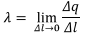Where Δq is the amount of charge contained in an infinitesimal element of length Δl.

The field due to the charged curve is given by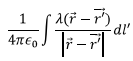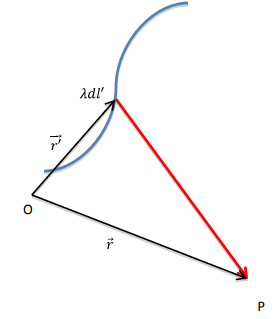Consider a charged surface with a surface charge density σ The charge density at a point on the surface is defined as the ratio of the amount of charge Δq contained in an infinitesimal surface element Δs at the point to the surface area of the element,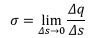The total charge contained in a surface is given by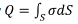Let an element of surface at the position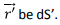The field due to a surface at a point P located at the position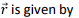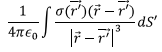Similarly, for a volume charge distribution, we define volume charge density as the ratio of the charge contained in an elementary volume at the point to the volume of the element,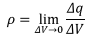The amount of charge contained in the volume is given by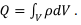The field at the point P due to a volume charge distribution is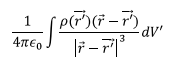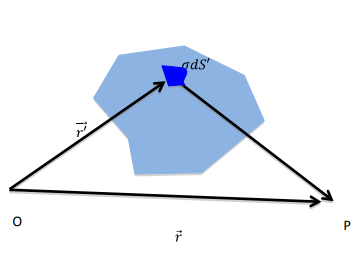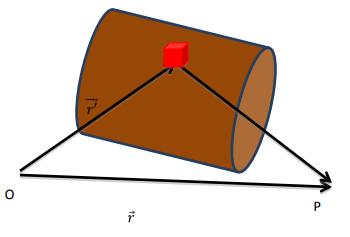Examples :

1. A line Charge : Consider a charged line of length L having a uniform linear charge density λ. We will place the line along with x axis with its centre at the origin. We will obtain an expression for the electric field at an arbitrary point P in the xy plane. Consider an element of the line of width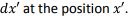The distance of the point P(x,y) from the charge element is given by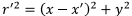and its position vector with respect to the element is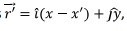The field at P(x,y) due to the element dx’ at (x’,0) is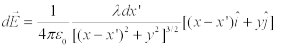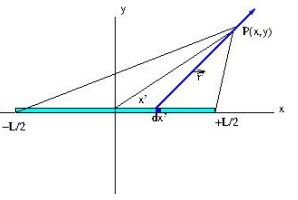The net field at P is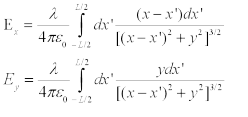These expressions cannot be evaluated in a closed form for an arbitrary point P. However, if the line charge is taken to be infinitely long, we can evaluate the integrals exactly. In this case, the xcomponent of the field becomes zero by symmetry.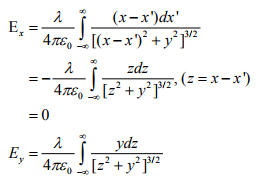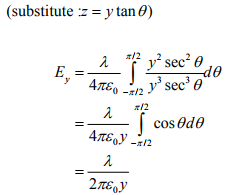2. Field due to a charged ring on its axis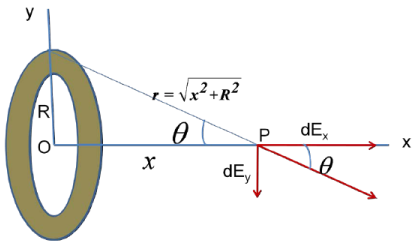The point P is taken along the x axis at a distance x from the centre of the charged ring. The ring is in the y-z plane. If we take an element on the ring, all such elements, by symmetry are located at the same distance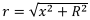from the point P. The field at P due to the element is along the radial direction from the element to the point P and can be resolved as shown. By symmetry the y-components would cancel.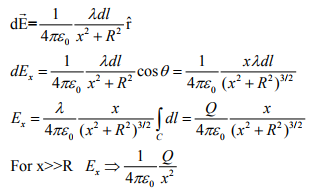Thus the field is directed along the axis. An interesting point to note is that for large distances, the field has the same form as that due to a point charge. This is to be expected as from such distances, the ring would look like a point.

Lines of Force :

The electric field is a vector field. We had earlier seen how vector fields are represented graphically. On a paper two dimensional vector fields are sketched by drawing arrows along the direction in which they point, the length of the arrow being proportional to the strength of the field. One can similarly represent these in 3 dimensions using packages such as Mathematica.

As we have seen the electric field is defined as the force experienced by a unit test charge, which is taken to be positive. Consider the field due to a positive charge. The field is clearly spherically symmetric since the force is central. The strength of the force on the test charge is Inverse square of the distance from the source. The arrows are directed away from the source because the force is repulsive. Experimentally, the direction of the arrow is the direction in which a positive test charge would move when placed in the vicinity of the source. Such representation of the electric field is known as the “line of force”. Reverse is true for the line of force due to a negative charge which would attract a test charge. The following figures show the lines of force due to a positive and a negative charge.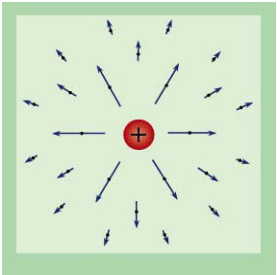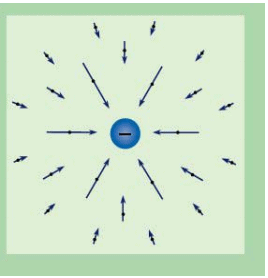We also show here the lines of force due to a pair of charges, one positive and the other negative. The lines would diverge from a positive charge (repulsive force, a source) and would converge into a negative charge (attractive force, sink)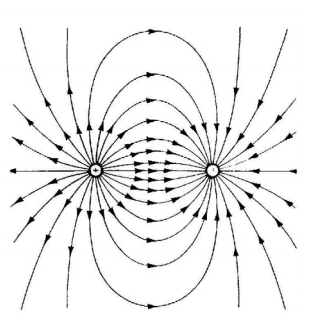The following figure shows the lines of force due to a pair of positive charges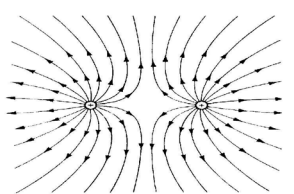Tutorial Assignment

1. Charges are kept on a circular dial so that 1 unit of charge is at the 1’O clock position, 2 units at 2’O clock position, and so on ending with 12 units of charge at the 12’Oclock ,position. Find the magnitude and the direction of the electric field at the centre of the dial.

2. A line charge of length L has a charge density λ. Find the electric field at a distance d along the perpendicular bisector of the line.

3. A particle of mass m having a charge q moves in a circular orbit with a speed v about an infinite line charge. Calculate the line charge density in terms of m,q and v.

4. A semi infinite line charge having a constant linear charge density lies along the x-axis from x = - ∞ to 0. Find an expression for the electric field at a point (0,y). Further show that the direction of the field makes an angle of 450 with the x axis, irrespective of the distance y.

5. A semi-circular arc is placed in the xy plane such that its diameter is along the x-axis and centre at the origin. The arc carries a linear charge density of constant magnitude λ which is positive in the first quadrant and negative in the second quadrant. Find the field at the centre.

Solutions to Tutorial Assignment

1. By symmetry, the resulting field is due to a charge of 6 units kept at positions 1,2,3,4,5 and 6.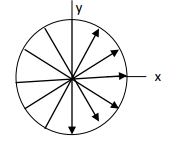Clearly, the y-components of the field as shown at 1’Oclock and 5’Oclock would cancel as will for 2’Oclock and 4’Oclock. Only y-component will be due to the 6’Oclock position and is given by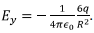X-components add up along the positive x-axis and is given by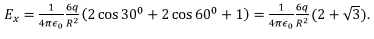2. Following Example 1, we take P(x,y) to be P(0,y) since P is along the perpendicular bisector. This makes Ex = 0 because the integrand is odd, which is to be expected by symmetry. We have,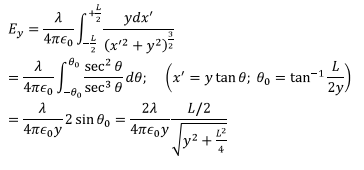3. Let the orbit radius be R. The centripetal force is provided by the electric force due to the line charge. Thus we have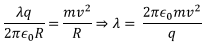4. In the example 1 for the line charge, let us place the charge along the x-axis from - ∞ to 0. we need to calculate the field at (0,y). The limits of integration over x’ are from - ∞ to 0. We get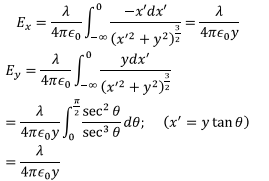Thus the angle with x axis is 450 .

5. Following the method outlined in Example 2, we take an element Rdθ on the arc (Let us consider only the positively charged quadrant), Since all the elements are at the same distance R from the centre of the circle, the field due to the first quadrant is as follows :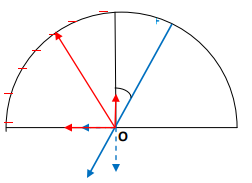The field due to the positive quadrant is shown in blue and that due to the negative octant in red. It is seen that the y- components cancel by symmetrically placed elements. The x-components add up and is directed along the negative x axis. We need to calculate the x-component due to the positive quadrant and multiply by 2 to take care of the negatively charged quadrant. The result is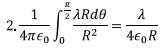Self Assessment Quiz

1. Consider a finite line charge of uniform charge density λ and of length L lying along the x-axis from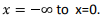Find the field at a point along the axis at x=d.

2. Find the point(s) on the axis of a charged ring where the electric field has maximum strength.

3. Find the electric field on the axis of a 600 arc of a circle of radius R with a uniform charge density λ. Leave the result in terms of an integral. In particular, find the field at the centre of the circle of which the arc is a part.

4. Determine the electric field at the centre of the base of a semicircle of radius R which has a charge density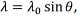where q is the angle made by an element on the semicircle with the base.

5. Two semi-infinite charged lines lie in the x-y plane making an angle of 1200 with each other. They are smoothly joined by an arc of a circle of radius R. The linear charge density is uniform throughout. Find the electric field at the centre of the circle of which the arc is a part.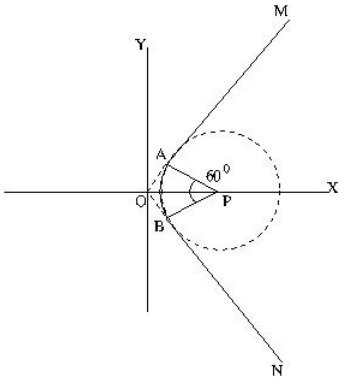Solutions to Self Assessment Quiz

1. Take an element pf length dx at a distance x from the origin. Since the point is at a distance d along the axis, the distance from this element is (x+d). The field is thus given by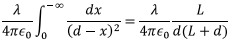2. From the expression derived in Example 2, the field on the axis of a charged ring at a distance x is given by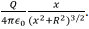Differentiate this expression and set the result to zero to obtain the maxima at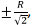the two signs indicate that the field becomes maximum to either side of the ring.

3. In doing this problem it is important to realize that the axis of an arc is in the same plane as the arc (unlike the case of a ring whose axis is perpendicular to the plane of the ring). Consider the field at a distance x from the centre of the circle. If we take an element of length Rdθ at an angle θ, the point P is at a distance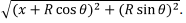The y-component of the field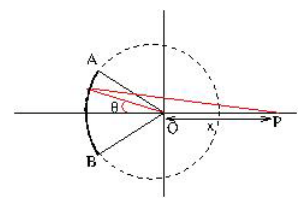cancels by symmetry. The x-component is given byThe field at the point O is obtained by taking x=0. It gives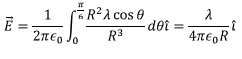4. Take an element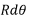on the semicircle at an angle θ with respect to x axis. The strength of the electric field at O due to the charge element is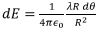directed radially outward. We can resolve this along x and y axes.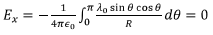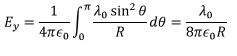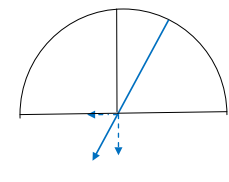5. We have seen in the Tutorial problem 4 that the field at a perpendicular distance y from the edge of a semi-infinite line has equal components perpendicular to the line and parallel to it (but in opposite direction), each component being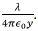In the figure below we show the field directions at P due to two semi-infinite wires, the directions shown in red is due to the line below the x-axis and that shown in blue is due to the wire above the x-axis. The angle between A1P and B1P is given as 600 and distance of P from each of the wires is R. Considering one of the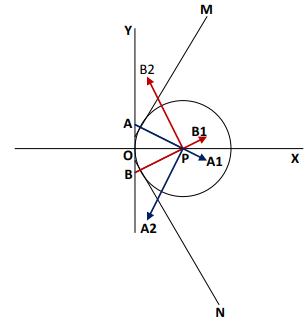wires (say BN), we resolve the fields along x and y directions :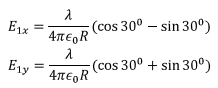If we consider the wire AM, we get similar expressions but the y-components are in the reverse to direction to that due to BN while the x components add up. The resulting contribution due to the two lines is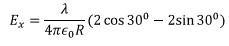To complete the problem we have to add the contribution due to the 600 arc. We have seen in Problem 3 that the field due the arc is along its axis which in this case is the x axis and has a magnitude given by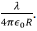Adding all contributions we have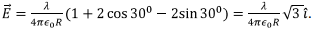The document Electric Field & Potential - Notes | Study Electromagnetic Fields Theory (EMFT) - Electrical Engineering (EE) is a part of the Electrical Engineering (EE) Course Electromagnetic Fields Theory (EMFT).
All you need of Electrical Engineering (EE) at this link: Electrical Engineering (EE)

## Electromagnetic Fields Theory (EMFT)

11 videos|46 docs|62 tests
 Use Code STAYHOME200 and get INR 200 additional OFF

## Electromagnetic Fields Theory (EMFT)

11 videos|46 docs|62 tests

Track your progress, build streaks, highlight & save important lessons and more!

,

,

,

,

,

,

,

,

,

,

,

,

,

,

,

,

,

,

,

,

,

;